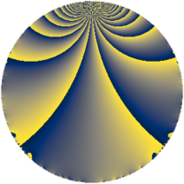# Properties

 Label 275.2.aLevel $275$ Weight $2$ Character orbit 275.a Rep. character $\chi_{275}(1,\cdot)$ Character field $\Q$ Dimension $16$ Newform subspaces $8$ Sturm bound $60$ Trace bound $3$

# Related objects

## Defining parameters

 Level: $$N$$ $$=$$ $$275 = 5^{2} \cdot 11$$ Weight: $$k$$ $$=$$ $$2$$ Character orbit: $$[\chi]$$ $$=$$ 275.a (trivial) Character field: $$\Q$$ Newform subspaces: $$8$$ Sturm bound: $$60$$ Trace bound: $$3$$ Distinguishing $$T_p$$: $$2$$

## Dimensions

The following table gives the dimensions of various subspaces of $$M_{2}(\Gamma_0(275))$$.

Total New Old
Modular forms 36 16 20
Cusp forms 25 16 9
Eisenstein series 11 0 11

The following table gives the dimensions of the cuspidal new subspaces with specified eigenvalues for the Atkin-Lehner operators and the Fricke involution.

$$5$$$$11$$FrickeDim
$$+$$$$+$$$+$$$3$$
$$+$$$$-$$$-$$$5$$
$$-$$$$+$$$-$$$6$$
$$-$$$$-$$$+$$$2$$
Plus space$$+$$$$5$$
Minus space$$-$$$$11$$

## Trace form

 $$16 q - q^{2} + q^{3} + 13 q^{4} - 2 q^{6} + 6 q^{7} - 3 q^{8} + 11 q^{9} + O(q^{10})$$ $$16 q - q^{2} + q^{3} + 13 q^{4} - 2 q^{6} + 6 q^{7} - 3 q^{8} + 11 q^{9} - 2 q^{11} + 18 q^{12} + 2 q^{13} - 8 q^{14} + 3 q^{16} - 12 q^{17} - 11 q^{18} + 8 q^{19} - 6 q^{21} + q^{22} - 3 q^{23} - 16 q^{24} + 22 q^{26} - 5 q^{27} + 8 q^{28} - 6 q^{29} + q^{31} - 7 q^{32} + q^{33} - 30 q^{34} - 17 q^{36} + 3 q^{37} + 4 q^{38} + 24 q^{39} - 2 q^{41} - 12 q^{42} + 14 q^{43} - 9 q^{44} - 30 q^{46} + 4 q^{47} - 4 q^{48} + 32 q^{49} - 2 q^{51} - 14 q^{52} - 4 q^{53} - 6 q^{54} - 12 q^{56} + 6 q^{58} - 25 q^{59} - 6 q^{61} + 22 q^{62} + 16 q^{63} - 13 q^{64} + 6 q^{66} + 15 q^{67} - 14 q^{68} - 37 q^{69} - 9 q^{71} - 39 q^{72} - 10 q^{73} + 24 q^{74} - 8 q^{76} + 6 q^{77} - 24 q^{78} + 22 q^{79} - 20 q^{81} - 30 q^{82} + 22 q^{83} - 64 q^{84} - 72 q^{86} - 32 q^{87} - 9 q^{88} - 21 q^{89} + 36 q^{91} + 22 q^{92} + 7 q^{93} + 64 q^{94} + 4 q^{96} + q^{97} + 7 q^{98} + 9 q^{99} + O(q^{100})$$

## Decomposition of $$S_{2}^{\mathrm{new}}(\Gamma_0(275))$$ into newform subspaces

Label Dim $A$ Field CM Traces A-L signs $q$-expansion
$a_{2}$ $a_{3}$ $a_{5}$ $a_{7}$ 5 11
275.2.a.a $1$ $2.196$ $$\Q$$ None $$-1$$ $$0$$ $$0$$ $$0$$ $+$ $+$ $$q-q^{2}-q^{4}+3q^{8}-3q^{9}-q^{11}-2q^{13}+\cdots$$
275.2.a.b $1$ $2.196$ $$\Q$$ None $$2$$ $$1$$ $$0$$ $$2$$ $+$ $-$ $$q+2q^{2}+q^{3}+2q^{4}+2q^{6}+2q^{7}+\cdots$$
275.2.a.c $2$ $2.196$ $$\Q(\sqrt{2})$$ None $$-2$$ $$0$$ $$0$$ $$4$$ $+$ $-$ $$q+(-1+\beta )q^{2}-2\beta q^{3}+(1-2\beta )q^{4}+\cdots$$
275.2.a.d $2$ $2.196$ $$\Q(\sqrt{5})$$ None $$-1$$ $$-3$$ $$0$$ $$-1$$ $-$ $-$ $$q-\beta q^{2}+(-1-\beta )q^{3}+(-1+\beta )q^{4}+\cdots$$
275.2.a.e $2$ $2.196$ $$\Q(\sqrt{13})$$ None $$-1$$ $$-1$$ $$0$$ $$-5$$ $+$ $+$ $$q-\beta q^{2}+(-1+\beta )q^{3}+(1+\beta )q^{4}-3q^{6}+\cdots$$
275.2.a.f $2$ $2.196$ $$\Q(\sqrt{13})$$ None $$1$$ $$1$$ $$0$$ $$5$$ $-$ $+$ $$q+\beta q^{2}+(1-\beta )q^{3}+(1+\beta )q^{4}-3q^{6}+\cdots$$
275.2.a.g $2$ $2.196$ $$\Q(\sqrt{5})$$ None $$1$$ $$3$$ $$0$$ $$1$$ $+$ $-$ $$q+\beta q^{2}+(1+\beta )q^{3}+(-1+\beta )q^{4}+(1+\cdots)q^{6}+\cdots$$
275.2.a.h $4$ $2.196$ $$\Q(\sqrt{3}, \sqrt{11})$$ None $$0$$ $$0$$ $$0$$ $$0$$ $-$ $+$ $$q+\beta _{1}q^{2}+(\beta _{1}-\beta _{2})q^{3}+(2+\beta _{3})q^{4}+\cdots$$

## Decomposition of $$S_{2}^{\mathrm{old}}(\Gamma_0(275))$$ into lower level spaces

$$S_{2}^{\mathrm{old}}(\Gamma_0(275)) \cong$$ $$S_{2}^{\mathrm{new}}(\Gamma_0(11))$$$$^{\oplus 3}$$$$\oplus$$$$S_{2}^{\mathrm{new}}(\Gamma_0(55))$$$$^{\oplus 2}$$